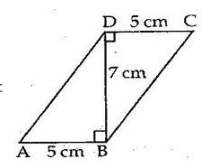# RS Aggarwal Class 9 Solutions Chapter 10 - Area

## RS Aggarwal Class 9 Chapter 10 - Area Solutions Free PDF

A quantity that measures the top shape, planar lamina and plane. The surface area is the area of a two-dimensional surface on a three-dimensional object. The unit of area is primarily concerned with the side of the length and they are primarily measured in square meters, square kilometers, square yards, square miles, etc. Some of the areas of different shapes are:

1. Quadrilateral Area
2. Triangle Area
3. Rectangular Area
4. Polygon Area
5. Area of a Circle
6. Area of an Ellipse
7. Surface Area
8. Fractals
9. Area of Calculus

## Download PDF of RS Aggarwal Class 9 Solutions Chapter 10 – Area

The RS Aggarwal Maths solutions are the most beneficial resources to cover the entire Class 9 maths syllabus in an efficient way. Class 9 Maths RS Aggarwal Solutions for Chapter 10 are created by subject experts in a simple language for better understanding of the students. All the solutions are prepared as per the latest syllabus of CBSE.

Q.1: In the adjoining figure, show that ABCD is parallelogram .Calculate the area parallelogram ABCD.Sol:### RS Aggarwal Class 9 Solutions Chapter 10 – Area

The RS Aggarwal Class 9 solutions will be very helpful for the students in case of clearing their doubts quickly and learning the most easy and convenient way to write paper during the examinations. The solutions of Class 9 maths provided here are solved with appropriate description. It contains all the necessary information and guidelines that a student is required to score well in their examination. It is the best resource for students to practice variety of questions from each and every topic.

AreaofΔABD=12